International Journal of Geomagnetism and Aeronomy
Vol. 1, No. 1, April 1998

# The method of calculation of the tide variations in the lower thermosphere from the UARS wind measurements

I. V. Karpov

Institute of Terrestrial Magnetism, Ionosphere, and Radio Wave Propagation, Troitsk, Moscow Region, Russia

### Abstract

From measurements of the wind horizontal components by the Upper Atmosphere Research satellite (UARS) in February 1992 the amplitudes and phases of the Hough functions for the diurnal and semidiurnal solar migrating tides are calculated. The calculations demonstrate that the structure of the semidiurnal tide in the lower thermosphere is mainly determined by the ( 2,2)  and (2,4)  tidal modes. At altitudes of 90-100 km the (2,3)  mode contribute significantly to the tidal structure. The (1,-1)  and (1,-2)  evanescent modes of the diurnal tide are responsible for a considerable portion of the tidal structure below 90 km. The calculated amplitudes of the tidal modes exceed the amplitudes of the corresponding modes measured by radars. The phases of the tidal modes determined for the zonal wind component as measured by the satellite agree with the results of radar observations. The amplitudes and phases of the diurnal and semidiurnal tides at middle latitudes are calculated using the mode structure determined from the satellite measurements. The calculated tidal amplitudes exceed the amplitudes obtained in radar observations. Good agreement with radar observations is obtained for the times of maximuma in both the diurnal and semidiurnal tides in the zonal component of the wind velocity.

### Introduction

Tides in the upper atmosphere are determined as global wave perturbations of the hydrodynamic parameters with periods rigorously equal to 24 h/n  ( n  is integer) are meant. The longitudinal and latitudinal variations of the tides are described by the zonal harmonics and tidal modes: the Hough functions which are solutions of the tidal Laplace equation. This definition makes it possible to consider the observed variations of atmospheric parameters with the periods, which correspond to the tidal disturbances, as a superposition of the tidal modes. Therefore, the problem of determination of tidal parameter variations in the atmosphere is reduced to determination of the amplitudes and phases of the Hough functions.

Radar observations of the horizontal wind components are usually used to determine the mode composition of the tides in the lower thermosphere and mesosphere. The methods used to calculate the amplitudes and phases of the Hough functions are based on analysis of the vertical structure of the observed parameters [ Fakhrutdinova and Ishmuratov, 1991; Forbes and Salah, 1991]. However, due to difficulties of providing continuous observations and to inhomogeneous distribution of the ground-based stations, the parameters of the tidal variations in the lower thermosphere are not adequately studied and need specification.

The use of satellite observations, for example the winds measured by UARS satellite, provides a significant expansion of the experimental data on the tides in this atmospheric region. To measure winds in the mesosphere and lower thermosphere, the HRDI instrumentation (High Resolution Doppler Imager) is installed on board the satellite and makes it possible to provide measurements at altitudes of50-115  km in the daytime and90  km at night. The detailed description of the HRDI and of its functioning on board UARS was given by Hays et al. . The orbit parameters provide measurements in the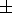72o  latitude band. During 24 hours the measurements cover all the longitudes. The results of observations of the wind tidal variations by the HDRI were presented by Burrage et al. [1995a, b\link2] and Hays et al. [1993, 1994]. It is worth noting that the procedure of extracting of the tidal variations used in the above papers needs accumulation of the data for several weeks of observations [ Burrage et al., 1995a]. It is because the UARS was slowly precessing along longitude (5o  per day) and so 36 days of observations were needed to cover all the LT moments [ Hays et al., 1993].

High horizontal resolution of the observations (500  km) made it possible to obtain such combinations of data, which provide determination of the mode composition of the tides from an analysis of the latitudinal structure of wind variations. The aim of this paper is to develop the method of determination of the amplitudes and phases of the migrating tidal modes from the latitudinal distributions of the wind components obtained in UARS measurements. We hope that such method of tidal mode parameter determination would make it possible to determine the global characteristics of the zonally averaged tidal variations for shorter observation period than it has been done by Burrage et al. [1995a, b\link2].

### Processing of the Experimental Data

Results of the observations of the horizontal wind components in the lower thermosphere on board the UARS satellite in February 1992 were presented by Hays et al. . During this observational period the satellite orbit parameters provided wind measurements in the latitude band from60o  in the northern hemisphere to40o  in the southern hemisphere. The experimental data were presented as zonally averaged values of the wind horizontal components at altitudes of 60-100 km at various LT moments.

Let us assume that the diurnal variations of the wind in the lower thermosphere are determined only by the tidal motions. The expression for the wind horizontal components may be written as

U(r, q, t)=U0(r, q)+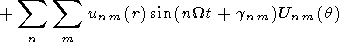(1)

Here r  and q  are the height and colatitude, respectively; t  is the local time; n  and m  are the indices of the tidal modes; U  is the observed value of wind velocity; Unm  are the tidal modes in terms of which the wind velocity components are expanded; anm  is the amplitude of the tidal mode; gnm  is the phase shift of the tidal mode; U0  is the daily mean value of the wind velocity component. Below writing the formulas we will omit the brackets with arguments of the functions.

In the most cases it is sufficient to take into account the diurnal (n=1)  and semidiurnal (n=2)  tides at altitudes of the lower thermosphere. The zonal averaging of the experimental data is taken into account in (1), so the formulas do not contain any dependence on longitude, and the indices of the tidal modes correspond to the solar migrating tides, because the tidal modes with other relation between the zonal wave numbers and frequencies disappear in the averaging process. The Unm  tidal modes, in terms of which the horizontal wind components are expanded, are related to the Hough functions by the relations of the classical tidal theory and satisfy the orthogonality condition:(2)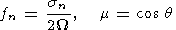Here sn = nW  is the frequency of the tidal perturbation; W  is the angular velocity of the Earth's rotation; Nnm  is a normalizing factor; and dml  is the Kroneker symbol. Determination of the mode composition is reduced to determination of the unknown amplitudes unm  and phases gnm . Using (2) one can obtain from (1)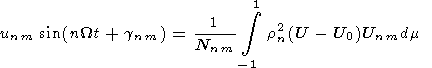(3)

Knowing the velocity components at various moments one can obtain from (3) a simple system of equations, solution of which determines the unknown amplitudes and phases:

unm=Gnm2(t1)+Gnm2(t2)-2Gnm(t1)Gnm(t2)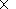(4)Here Gnm(t1)  and Gnm(t2)  are the values of the right-hand sides of (3) for the t1  and t2  moments. The main uncertainties in determination of the unknown amplitudes and phases of the tidal modes are due to a limited latitudinal interval for which the experimental data are available, and also to a character of the latitude variations of the kernel in (3). For the considered period of observations on board the UARS satellite there are no data for latitudes above 60o  in the northern hemisphere and 50o  in the southern hemisphere. The error in phase and amplitude determination of the tidal modes due to a lack of measurements at high latitudes is negligible for all semidiurnal solar migrating tides, because the kernel in (3) rapidly decreases to the poles. Latitudinal variations of the kernel in (3) for the diurnal tidal modes are of a more complicated character and so the calculation errors for some modes may be significant. Unlike the methods used to determine the mode composition of the tides by the ground-based radar observations, in the method suggested there is no requirement to assume the set of Hough functions in advance to approximate the tidal perturbations, and the inputs of various tidal modes into the observed variations of the parameters are calculated directly by (2)-(4).

### DiscussionThe results of calculations of the amplitudes and local times of the maximum of the geopotential semidiurnal tidal modes at altitudes of 70-110 km are presented in Figure 1. The solid lines show the tidal mode parameters, determined from the observations of the wind meridional component, the dashed lines indicate the parameters determined from the zonal component observations. Unfortunately, a small volume of the experimental data presented by Hays et al.  prevents determination of the daily mean wind directly from the UARS observations. This can be helped if the measurements for a long observational period or modern models of the mean parameters of the upper atmosphere are used. The results in Figure 1 were obtained without taking into account the daily mean wind (U0 = 0) . Such calculations contain some uncertainty in determination of the amplitudes and phases of the tidal modes and this uncertainty may be significant if the zonal wind component is used. The results obtained (bearing in mind a small volume of the experimental data and neglecting by the mean wind) are of an illustrative character and merely demonstrate capacities of the method of tidal mode composition determination based on an analysis of the wind distribution mode structure. The tidal perturbations of the geopotential in Figure 1 were recalculated from the corresponding perturbations of the wind components using the relations of the classic tidal theory. Deviations from the values obtained due to the errors in the satellite measurements of the wind (5  m s -1 ) do not exceed 200 m and about 2 h in the determination of the amplitude of the geopotential perturbations and the time of the tidal mode maximum, respectively. This estimate apparently is strongly overestimated and obtained from (2)-(4) for the case when the wind velocity does nor depend on latitude and is equal to the measurement error. One can see that the parameters of the tidal modes determined by different wind components differ significantly, but at altitudes of about 90 km their amplitudes are fairly close to each other (Figure 1a). The symmetric tidal modes, (2,2)  and (2,4) , which tend to increase in amplitude above 100 km, prevail within the entire height interval. The asymmetric (2,3)  mode, the amplitude of which at an altitude of about 90 km exceeds the amplitudes of the symmetric tidal modes, contributes considerably to the structure of the semidiurnal tide at 90-100 km. The differences in times of maximum of the tidal modes (Figure 1b) determined by different components of the wind velocity are fairly strong, although the tendencies in vertical profile variation above 90 km coincide. Circles in Figure 1 show the parameters of the tidal modes obtained in theoretical and experimental studies at an altitude of 97 km. The open circles show the mode structure of the semidiurnal tide based on the Forbes and Vial  results of numerical simulation for spring equinox. The closed circles show parameters of the tidal modes obtained by Forbes and Salah  in the radar measurements of the wind in the course of the LTCS-1 Program in September 1987. Comparison of these results to the mode structure determined by the UARS measurements is in some sense verified by the fact that the considered observational period is close to equinox. One can see in Figure 1a that the amplitudes of the geopotential perturbations obtained in this paper significantly exceed other results [ Forbes and Salah, 1991].

It is worth noting that a significant increase of the (2,3)  mode in comparison with the main symmetric modes, (2,2)  and (2,4) , is found in the radar observations of Forbes and Salah . The same relationship between the inputs of various tidal modes is typical for our results. It can be seen in Figure 1b that at an altitude of about 97 km the phases of the tidal modes obtained in the radar observations at autumn equinox agree well with the phases determined by the measurements of the zonal wind component, and the phases of the tidal modes measured by Forbes and Vial  are closer to the phases determined by the measurements of the meridional wind component. Such a difference in the tidal mode phases can not be explained by a single reason. It is widely known that the equinox periods are characterized by a rapid change of the tidal phases [ Manson et al., 1989], therefore coincidences, as well as differences, based on a comparison to a small amount of data may be occasional. Moreover, the amplitudes and phases of the tidal modes determined from the ground-based observations depend on the number of stations used for the analysis, their geographic position and the instrumentation used [ Forbes and Salah, 1991]. Due to that, the UARS wind observations have a considerable advantage in determination of the tide mode composition as compared with ground-based measurements. The amplitude and phase differences of the semidiurnal solar migrated tides determined from the measurements of the zonal and meridional wind components observed on board the UARS are apparently due to the fact that in the calculations performed no daily mean wind has been taken into account. To evaluate influence of the mean flow on the semidiurnal tide mode structure. a calculation has been performed using the zonal component of the mean wind at altitudes of 70-80 km from the CIRA-65 empirical model. The results are shown in Figure 1 by the dashed line. Figure 1 demonstrates that taking into account of the daily mean wind alters significantly parameters of the principal tidal modes (2,2)  and (2,3)  and only slightly influence other tidal modes.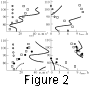The mode structure obtained by satellite measurements was used to reconstruct the semidiurnal tidal parameters at middle latitudes. The amplitudes and phases of the tidal modes determined from observations of the meridional and zonal components of the wind velocity (Figure 1) were used to calculate the tides in these components. Figure 2 shows the results of calculations for the latitude of 50o  and also the results of radar observations in February. The measurements at various stations were presented by Manson et al.  and are shown in Figure 2 in the following way: closed circles - Garchy (47o N, 3o E), open circles - Colm (52o N, 15o E), squares - Saskatoon (52o N, 107o W).

The tidal amplitudes based on the UARS measurements exceed those observed, especially for the zonal velocity component below 90 km (Figure 2b). Tidal amplitudes in the meridional velocity component below 100 km agree with the Saskatoon data (Figure 2a). The tide amplitudes in the horizontal wind components measured at Colm are significantly lower the ones obtained by us. The time of tide maximum derived from the observations of the velocity meridional component (Figure 2a) differs significantly from the observational data, whereas the calculated time of tidal maximum in the zonal wind component agrees well with the observations at altitudes of 80-100 km (Figure 2b).

The discrepancies in the parameters of the observed and calculated tides may be due to the difference in methods used to determine the wind in the lower thermosphere from satellite and radar data. It should be noted that the UARS wind observations show that the wind velocity measured by HRDI exceeds the velocities obtained by the ground-based radar measurements [ Burrage et al., 1996]. Evidently, the question of determination of the wind in the lower thermosphere by ion drifts measured in radar observations and by Doppler measurements of the molecular oxygen spectra on board the UARS satellite requires special consideration. Comparison of the results obtained in this paper with the characteristics of the semidiurnal tides derived from the HRDI measurements for two years of UARS observations [ Burrage et al., 1995b] demonstrates a satisfactory agreement for the amplitude of the wind meridional component and the time of a tide maximum in the wind zonal component. At 95 km, the amplitude of the velocity meridional component and the moment of the maximum of the velocity zonal component are30  m s -1  and8  h, respectively [ Burrage et al., 1995b]. In our calculations we obtained these parameters to be40  m s -1  and8  h. The differences in the amplitude of the velocity zonal component and the moment of the maximum are rather strong. Apparently, the principal source of these differences is the fact that we did not take into account the daily mean wind, which influences the tide mode composition (see Figure 1). The amplitudes and phases of the semidiurnal tidal modes obtained with allowance for the daily mean zonal wind (dashed lines in Figure 1) were used to calculate the semidiurnal tide in the zonal wind component. The results are presented in Figure 2b by the dashed line and demonstrate that use of the specifies mode composition of the tides improves the agreement of the calculated and observed tide parameters. Thus, calculating the tidal mode structure from observations of the lower thermospheric wind, one should take into account the input of the wind daily mean component. In the calculation methods of the tide mode structure based on analysis of the vertical profiles of the wind [ Fakhrutdiniva and Ishmuratov, 1991; Forbes and Salah, 1991] obtained in radar observations, the input of the daily mean wind should be taken into account directly in the equations for the vertical structure of the modes.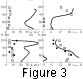Similar calculations are performed to determine parameters of the diurnal tidal modes. It was assumed in calculations by (2)-(4) that the solar diurnal tides are determined by inputs of the (1,1) , (1,-1 ), (1,-2 ), (1,4) , and (1,3)  tidal modes. The calculations performed demonstrate that the diurnal tide structure is mainly determined by the (1,1)  mode. Some input into the diurnal variations below 90 km is also made by the (1,-1 ) and (1,-2 ) modes, their amplitudes decreasing rapidly with height. It is worth noting that the error of the method of mode input calculation for the (1,-1 ) and (1,-2 ) modes in the UARS observational period in question is rather high (50%)  and thus the result obtained is merely of a qualitative nature. In the same way as for the semidiurnal tides, differences in tidal mode amplitude and phase, determined from different wind components, appear. Figure 3 shows the amplitudes and the time of maximum of the diurnal tide in the velocity horizontal components at an latitude of 50o  calculated by the tidal mode parameters determined from the satellite measurements. The results of radar observations of the diurnal tides for February by Manson et al.  are also shown in Figure 3. The indexing is the same as in Figure 2. It should be kept in mind that the comparison of our results with the results obtained earlier is of a qualitative character. Moreover, the tide observations show significant interannual variation of the diurnal tide amplitudes (see Burrage et al. [1995b]). It can be seen that the amplitudes of the diurnal tide calculated in this paper exceed significantly the observed values. A significant depletion of the diurnal tide amplitude is seen above 90 km, which is due to the dissipative processes in the thermosphere. The time of tidal maximum determined for the meridional wind component differs significantly from that observed (Figure 3a). However, the tidal phase calculated for the wind zonal component agrees well with the observations above 80 km (Figure 3b). Possible reasons of such discrepancies between our results and observations were discussed above while analyzing the semidiurnal tides.

### Conclusion

A new method to determine parameters of the solar diurnal and semidiurnal tides in the mesosphere and lower thermosphere from observations of the horizontal wind components on board the UARS satellite is suggested.

The method is based on an assumption that the temporal variability of the global distribution of parameters in this atmospheric region is governed by propagation of the solar migrating tides. The UARS measurements cover a wide latitudinal range and make it possible to determine the mode structure of the solar tides in the atmosphere, using the properties of orthogonal modes. Our method is a development of the idea of studying the tidal structure from the UARS observations applied to the diurnal tides by Hays .

The develop procedures of calculation of the tidal mode input and diurnal and semidiurnal mode parameters are applied to a limited set of the UARS observations in February 1991. The results of the calculations lead to the following conclusions:

1. The amplitudes and phases of the tidal modes determined by observations of the zonal and meridional components of the wind differ. Parameters of the above modes depend significantly on the daily mean wind. One of possible reasons of the difference in parameters of the tidal modes determined by different wind components may be related to not taking into account this factor in the above described calculations.

2. The semidiurnal tide structure is mainly determined by the (2,2) and (2,4)  modes. The calculations reveal a significant increase of the mode (2,3)  amplitude between 90 and 100 km. An increase of the input of this mode during equinoxes was also detected in the radar observations by Forbes and Salah . The diurnal tide structure is mainly determined by the (1,1)  tidal mode.

3. Calculations of the midlatitude tidal parameters using the mode structure determined by the measurements on board UARS satellite show that the calculated tide amplitudes exceed the amplitudes obtained in the radar observations, especially for the diurnal tide. The times of tidal maxima in the meridional wind component differ significantly from the observational results, whereas the times of maxima of the diurnal and semidiurnal tides in the zonal component of the wind agree well with the observational data.

The results of the preliminary study show that the UARS measurements open broad possibilities to explore the tide morphology in the mesosphere and lower thermosphere.

The UARS measurements provide a possibility to improve significantly the accuracy of determination of the tidal mode structure, which is very important for theoretical modeling of the upper atmosphere. Attracting to tide analysis observations of other atmospheric parameters on board UARS (for example, the temperature) would improve tidal parameter determination and eliminate the uncertainties arising when the wind measurements are used.

#### Acknowledgments

This work was supported by the Russian Foundation for Basic Research (project 94-05-17433).

### References

Burrage, M. D., et al., Long-term variability in the solar diurnal tide observed by HRDI and simulated by the GSWM, Geophys. Res. Lett., 22, 2641, 1995a.

Burrage, M. D., et al., Latitude and seasonal dependence of the semidiurnal tide observed by HRDI, J. Geophys. Res., 100, 11,313, 1995b.

Burrage, M. D., et al., Validation of the mesosphere and lower thermosphere winds from the High Resolution Doppler Imager on UARS, J. Geophys. Res., 101, 10,365, 1996.

CIRA 1965, COSPAR Working Group IY, Amsterdam, 1965.

Fakhrutdinova, A. Ya., and R. A. Ishmuratov, Seasonal variations of the mode input into the semidiurnal tidal motions in the midlatitude lower thermosphere, Geomagn. Aeron., 31 (5), 904, 1991.

Forbes, J. M., and J. E. Salah, Mesosphere-thermosphere tidal coupling during the September 21-25, 1987, LTCS-1 campaign, J. Geophys. Res., 96 (A2), 1135, 1991.

Forbes, J. M., and F. Vial, Monthly simulations of the solar semidiurnal tide in the mesosphere and lower thermosphere, J. Atmos. Terr. Phys., 51, 649, 1989.

Forbes, J. M., R. G. Roble, and C. G. Fesen, Acceleration, heating and composition mixing of the thermosphere due to upward propagating tides, J. Geophys. Res., 98 (A1), 311, 1993.

Hays, P. B., et al., Remote sensing of mesosphere winds with the High Resolution Doppler Imager, Planet. Space Sci., 40 (12), 1599, 1992.

Hays, P. B., et al., The High Resolution Doppler Imager on the Upper Atmosphere Research Satellite, J. Geophys. Res., 98, 10,713, 1993.

Hays, P. B., et al., Observations of the diurnal tide from space, J. Atmos. Sci., 51, 3077, 1994.

Manson, A. H., et al., Climatologies of semidiurnal and diurnal tides in the middle atmosphere (70-110 km) at middle latitudes (40-55o), J. Atmos. Terr. Phys., 51 (7/8), 579, 1989.

Load file for printing and local use. This document was generated by TeXWeb (Win32, v.1.0) on March 25, 1998.